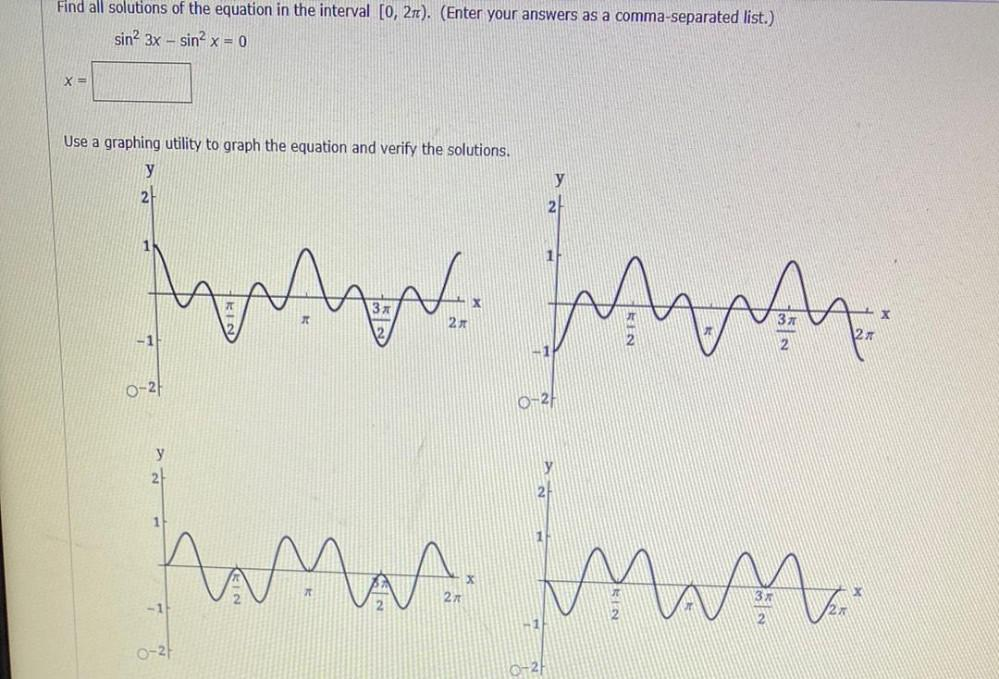Question:

# Find all solutions of the equation in the interval [0, 271). (Enter your answers as a comma-separated list.) sin? 3x - sin? x =Find all solutions of the equation in the interval [0, 271). (Enter your answers as a comma-separated list.) sin? 3x - sin? x = 0 X= Use a graphing utility to graph the equation and verify the solutions. y y 2 1 21 2x 11 0-24 0-21 Ants for hann inn y 2 y 2. 1 X 27 -1 2X -1 0-27